# Kinetics images

Kinetics

Up until now, we have only looked at what everything is made of -- matter.

Now we will look at its movement.

The study of movement is called kinetics.

You will learn the basic laws and formulae for motion as well as simple machines.

You will explore this content through several building projects.

You will also focus more deliberately on note-taking.

What is energy?

Energy

Our class's definition of energy:

Two types of energy:

Kinetic vs Potential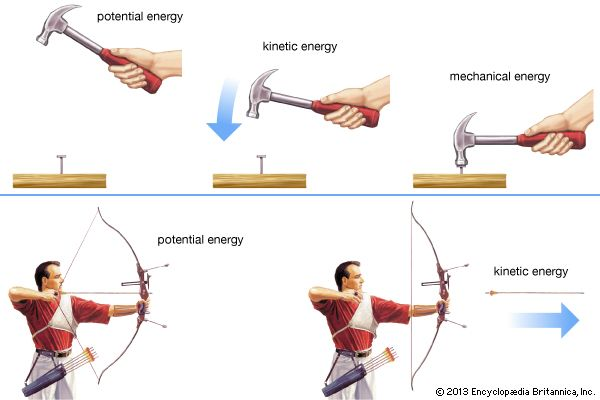Define potential energy:

Define kinetic energy:

Put these definitions in your notebook.

Energy cannot be created or destroyed, only change forms (except through E=mc2, but that is for another time).

It is important to note that we cannot escape friction which resists all motion and slows everything down.

Now let us try to minimize friction and convert energy forms by building potential energy cars!Sir Isaac Newton

Everyone knows about Newton's law of gravity, right?

Well, everyone knew that things fall toward Earth.  Everyone knew that what went up, must come down.  The breakthrough that Newton came up with was this: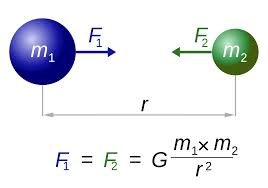This says that everything has gravity!

It also says that the force of gravity felt is a mutual force (you are pulling the Earth upwards right now).

It also says that the force of gravity is directly proportional to the masses of the two objects and inversely proportional to the distance between them.  A bigger object has more gravity.

This was revolutionary!  Newton could calculate the mass of something based on its gravity or the gravity of something based on its mass.  And he proved that everything behaves this way: people, planets, galaxies, etc.

In your notes, please record that gravity is proportional to mass and inversely proportional to distance.

Everything accelerates here on Earth at roughly the same rate (9.8 m/s2) until it hits its terminal velocity (this is based on its shape).  That means that a bowling ball and a feather both fall at the same rate...until the shape of the objects experience friction (drag) with the air and slow it down.

One more thing of note on gravity while we are here is about center of gravity.  Let's look at this...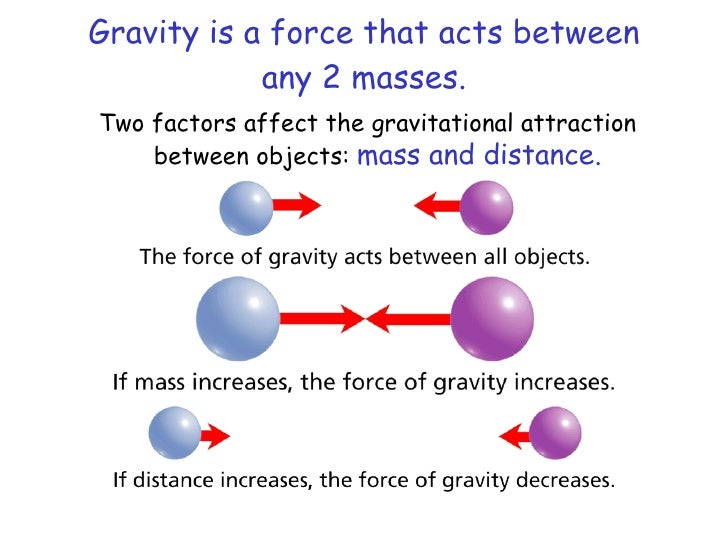Newton also discovered and clarified three laws of motion that govern everything you experience (not atoms or galaxies, we need Einstein's theory of special relativity for that).  You will need to write all three of these laws in your notebook.

Law #1:  Law of inertia.

An object in motion will stay in motion and an object at rest will stay at rest until acted upon by an outside forceNewton's second law: Law of force

F = MxANewton's third law: Law of reaction

For every action there is an equal and opposite reaction.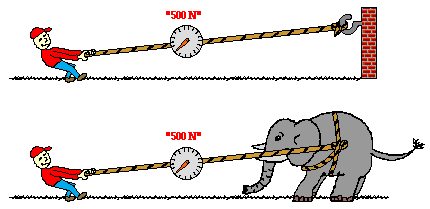Formulae:
Speed = distance / time
Velocity = speed in a direction
Acceleration = velocity / time
Momentum = mass x velocity
Force = mass x acceleration
Work = force / distance
Power = work / time

Look at the formula for work.  Simple machines can generate tremendous force by altering the distance moved.

Work into the simple machine = work out of it, so:
Force in x Distance in = Force out x Distance out

This means that if you exert 10 Newtons of Force over 100 meters while the other end of the simple machine moved only one meter, it would move with 1000 Newtons of force (100 times what you put in!).

Scientists agree that there are 6 simple machines: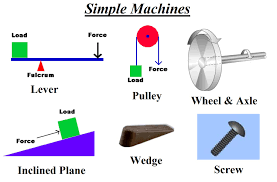Lever: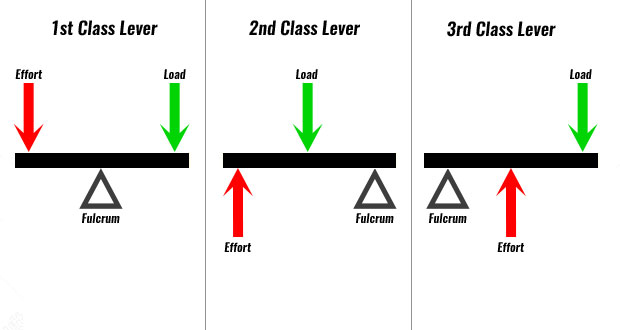Pulley: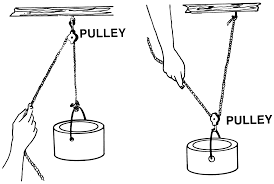Are you ready for this test?